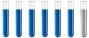## pH determination problems

Chemistry and homework help forum.

Organic Chemistry, Analytical Chemistry, Biochemistry, Physical Chemistry, Computational Chemistry, Theoretical Chemistry, High School Chemistry, Colledge Chemistry and University Chemistry Forum.

Share your chemistry ideas, discuss chemical problems, ask for help with scientific chemistry questions, inspire others by your chemistry vision!

Please feel free to start a scientific chemistry discussion here!

Discuss chemistry homework problems with experts!

Ask for help with chemical questions and help others with your chemistry knowledge!

Moderators: expert, ChenBeier, Xen

Dhamnekar Winod
Sr. Staff MemberPosts: 149
Joined: Sat Nov 21, 2020 10:14 am
Location: Mumbai[Bombay],Maharashtra State,India

### pH determination problems

Determine whether each of the following 1 M solutions is acidic, basic, or neutral.
a.NaHS
b.NaBr
c.NH4Ac (remember Ac = acetate)
d.KNO2
e.KHSO3
f.NaH2PO4

I am working on these questions.
Last edited by Dhamnekar Winod on Sat Apr 03, 2021 7:41 am, edited 3 times in total.
Any science consists of the following process. 1) See 2)Hear 3)Smell,if needed 4)Taste, if needed 5)Think 6)Understand 7)Inference 8)Take decision [Believe or disbelieve, useful or useless, true or false, cause or effect, any other criteria]
ChenBeier
Distinguished MemberPosts: 750
Joined: Wed Sep 27, 2017 7:25 am
Location: Berlin, Germany

### Re: pH determination problems

What are strong acid, base and weak acid, base. What are the corresponding species in every example?
Dhamnekar Winod
Sr. Staff MemberPosts: 149
Joined: Sat Nov 21, 2020 10:14 am
Location: Mumbai[Bombay],Maharashtra State,India

### Re: pH determination problems

All the 1 M aqueous solutions of the substances in the questions a, b, c, d, e, f are acidic.
Any science consists of the following process. 1) See 2)Hear 3)Smell,if needed 4)Taste, if needed 5)Think 6)Understand 7)Inference 8)Take decision [Believe or disbelieve, useful or useless, true or false, cause or effect, any other criteria]
ChenBeier
Distinguished MemberPosts: 750
Joined: Wed Sep 27, 2017 7:25 am
Location: Berlin, Germany

### Re: pH determination problems

No
A. Alkaline, B. Neutral C. Neutral, D. Alkaline, E. Acidic, F. Acidic

Explain why.: in A compare NaOH and H2S
The same in the other questions
Dhamnekar Winod
Sr. Staff MemberPosts: 149
Joined: Sat Nov 21, 2020 10:14 am
Location: Mumbai[Bombay],Maharashtra State,India

### Re: pH determination problems

You are correct. Answer for question a is alkaline. Following is my chemistry work.

I computed the pH of 1.0 M solution of NaHS as follows:- NaHS + H2O ⇌ NaOH + H2S

$$K_a of HS = 1.2e-13 = \frac{[NaOH])[H_2S]}{[1.0M]}$$ Concentration of H2S is =$$\sqrt{1.2e-13}=[3.46e-7]$$ We got concentration of H2S. Ionization of H2S is

H2S ⇌ H⁺ + HS

$$K_a of H_2S = 8.9e-8 = \frac {[H^+][1.0M ]}{[3.46e-7]}=3.08e-13$$ Hence pH of 1.0 M solution of NaHS=-log(3.08e-13)=12.51.

Hence the solution is alkaline.

Is my computation of pH correct?
Any science consists of the following process. 1) See 2)Hear 3)Smell,if needed 4)Taste, if needed 5)Think 6)Understand 7)Inference 8)Take decision [Believe or disbelieve, useful or useless, true or false, cause or effect, any other criteria]
ChenBeier
Distinguished MemberPosts: 750
Joined: Wed Sep 27, 2017 7:25 am
Location: Berlin, Germany

### Re: pH determination problems

Yes correct
Dhamnekar Winod
Sr. Staff MemberPosts: 149
Joined: Sat Nov 21, 2020 10:14 am
Location: Mumbai[Bombay],Maharashtra State,India

### Re: pH determination problems

Your answer to question e for pH of 1 M solution of KHSO3(Potassium Hydrogen Sulfite) is acidic.
But my pH computation work result is different.

KHSO3 + H2O ⇌ H2SO3 + KOH

$$K_a = \frac{K_w}{K_b}= \frac{1e-14}{1.5e-2}=\frac{x\cdot x}{1 M}= 6.66e-13,x= 8.16e-7,pOH=6.088,pH=7.911$$ So, the 1 M solution of KHSO3 is alkaline.

What is wrong with this pH computation?
Any science consists of the following process. 1) See 2)Hear 3)Smell,if needed 4)Taste, if needed 5)Think 6)Understand 7)Inference 8)Take decision [Believe or disbelieve, useful or useless, true or false, cause or effect, any other criteria]
ChenBeier
Distinguished MemberPosts: 750
Joined: Wed Sep 27, 2017 7:25 am
Location: Berlin, Germany

### Re: pH determination problems

Used wrong formula H2SO3 is a middle strong acid. pKa1 is 1.8 pka2 is 7.

pH =( pk1 + pk2)/2

pH =(1.8 +7)/2 = 4.4